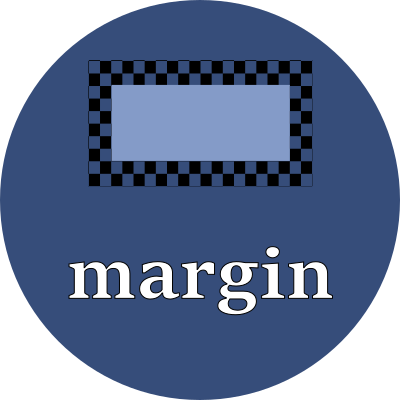# marginUse this property to specify margin-top, margin-right, margin-bottom, and margin-left.

## Property values

This property is used to set each of the four margin properties, which may also be set one by one:

• margin-top
• margin-right
• margin-bottom
• margin-width

This property expects anywhere from one to four values, with their interpretation as follows:

number of values sides effected
1 value Sets all four sides: top, right, bottom, left
2 values 1st value: top and bottom margins
2nd value: right and left margins
3 values 1st value: top margin
2nd value: right and left margins
3rd value: bottom margin
4 values 1st value: top margin
2nd value: right margin
3rd value: bottom margin
4th value: left margin

Use the `auto` keyword to let the browser determine how much margin to apply. When the left and right margins are both set to `auto`, the element is centered within its parent.

Use any of these font-relative typographic units to set the size of the margin:

 em em The `font-size` of the current font rem relative em The `font-size` of the element lh line height The `line-height` of the current font rlh relative line height The `line-height` of the element cap cap height The nominal height of the current font's capital letters ex "x" The height of the current font's "x" glyph ch "0" The advance measure (the width) of the current font's "0" glyph ic ideograph count The advance measure (the width) of the current font's "水" ideograph

Or use any of these fixed size units which are independent of the current font:

 in inch A size equal 96px px pixels A size equal to 1/96 inch pt point A size equal 1in/72 = 1.33px pc pica A size equal 1in/6 = 12pt = 16px cm centimeter A size equal to 96px/2.54 = 37.8px mm millimeter A size equal to 96px/25.4 = 3.8px Q quarter millimeter A size equal to 96px/1016 = 0.94px

## Examples

style > whitespace > marginMargin all-in-one spec